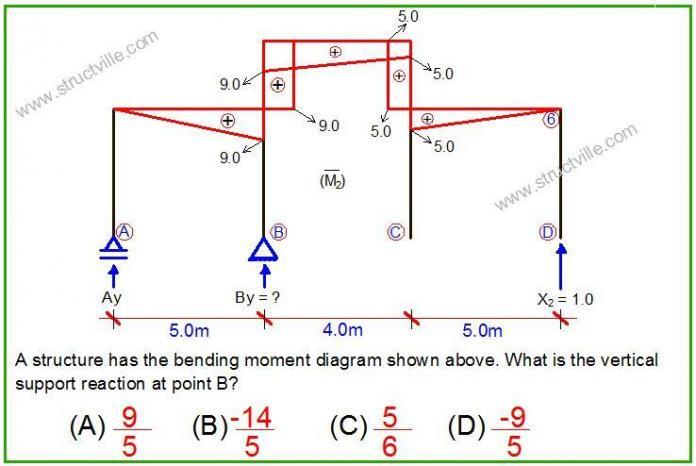# Question of the Day (04/06/2018)Structville daily questions
From now henceforth, Structville will be publishing daily questions on different aspects of civil engineering. You are expected to enter your response in the comment section. At the end of every week, exceptional participants will stand a chance to win some gifts. This exercise is open to participants all over the world. Happy new month to you all.

Today’s Question
What is the vertical support reaction at point B of the frame?

Thank you for participating in exercise today, remember to enter your answer in the comment section. The main aim of this exercise to stimulate knowledge of structural analysis on the internet in a fun and exciting way. We are always happy to hear from you, so kindly let us know how you feel about Structville.

E-mail: info@structville.com
WhatsApp: +2347053638996

You can also visit Structville Research for downloads of civil engineering materials.

STRUCTVILLE REINFORCED CONCRETE DESIGN MANUAL
We have this very affordable design manual available…

Do you want to preview the book, click PREVIEW

1. Sum of Moment respect point (A)= By x 5m + 1.0 x 14m = 0 then By = -1.0 x 14 / 5 = – 2.8kN = (-14/5) or if you look at the first triangular bending moment from A to B which is equal to 9.0kNm and divide it for the lever arm which is 5m it is possible to find the reaction Ay = 1.8kN then for the equilibrium of the vertical forces let's say: Ay=1.8kN + By + X2=1.0kn = 0 then By= -2.8 or (-14/5), hence B) is correct.

2.Ajit Kumar Mohanty

Total Reaction, Ay + By = – 1 kN (let's say Equation 1)

Ay x 5 = 1 x 9
Ay = 9/5 kN

Apply the value of Ay in Equation 1, we have

By = -1 – (9/5) = -14/5 kN

B) -14/5

5*Ay=9
Ay=9/5
sum of vertical forces =0
Ay+By+1=0
By=-1-Ay
=-1-9/5
=-14/5

4. (B) -14/5

5. A) -9/5

6. By = ((Mab-Mba)/Lab) + ((Mcb-Mbc)/Lbc))
= ((0-9)/5)) + ((5-9)/4)
=-9/5 + -1
=-9/5+-5/5
=-14/5

7.Luis Miguel García

-14/5

If you calculate moment from Point A you get he equation B*5 + (1)*14=0
And then you get B=-14/5